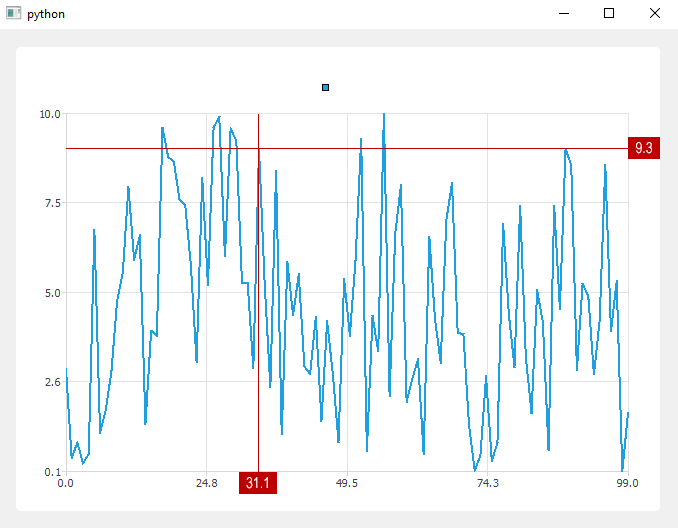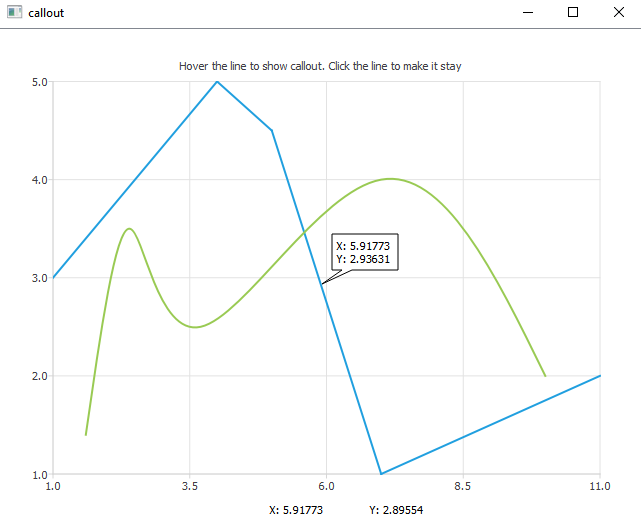# How add crosshair QtChart (with signed values)?

• Task: There is a chart (QtChart). Code:

``````from random import uniform
import sys

from PyQt5.QtWidgets import QApplication, QMainWindow, QVBoxLayout, QWidget
from PyQt5.QtChart import QChart, QChartView, QLineSeries

class Window(QMainWindow):
def __init__(self):
super().__init__()
self.setGeometry(100, 100, 680, 500)

series = QLineSeries()
for i in range(100):
series.append(i, uniform(0, 10))

chart = QChart()
chart.createDefaultAxes()
chartview = QChartView(chart)

central_widget = QWidget()
self.setCentralWidget(central_widget)
lay = QVBoxLayout(central_widget)
self.setMouseTracking(True)

def mouseMoveEvent(self, event):
mouse_x = event.x()
mouse_y = event.y()

if __name__ == "__main__":
App = QApplication(sys.argv)
window = Window()
window.show()
sys.exit(App.exec_())
``````

It is necessary: Add crosshair with signed values to the chart (QtChart).What is at the moment:

1. Thanks to the example https://stackoverflow.com/questions/41688668/how-to-return-mouse-coordinates-in-realtime, there is an understanding of how to get the mouse coordinates (code updated).
2. Unfortunately, we didn’t succeed in making any progress. An example was found in the chart documentation (QT) with similar functionality https://doc.qt.io/qt-5/qtcharts-callout-example.html (not quite what you need), but the main problem is that the code is written in C++.Below is the code in C++ (if it helps):

``````#include "callout.h"
#include <QtGui/QPainter>
#include <QtGui/QFontMetrics>
#include <QtWidgets/QGraphicsSceneMouseEvent>
#include <QtGui/QMouseEvent>
#include <QtCharts/QChart>

Callout::Callout(QChart *chart):
QGraphicsItem(chart),
m_chart(chart)
{
}

QRectF Callout::boundingRect() const
{
QPointF anchor = mapFromParent(m_chart->mapToPosition(m_anchor));
QRectF rect;
rect.setLeft(qMin(m_rect.left()+20, anchor.x()));
rect.setRight(qMax(m_rect.right(), anchor.x()));
rect.setTop(qMin(m_rect.top(), anchor.y()));
rect.setBottom(qMax(m_rect.bottom(), anchor.y()));
return rect;
}

void Callout::paint(QPainter *painter, const QStyleOptionGraphicsItem *option, QWidget *widget)
{
Q_UNUSED(option)
Q_UNUSED(widget)
QPainterPath path;

QPointF anchor = mapFromParent(m_chart->mapToPosition(m_anchor));
if (!m_rect.contains(anchor)) {
QPointF point1, point2;

// establish the position of the anchor point in relation to m_rect
bool above = anchor.y() <= m_rect.top();
bool aboveCenter = anchor.y() > m_rect.top() && anchor.y() <= m_rect.center().y();
bool belowCenter = anchor.y() > m_rect.center().y() && anchor.y() <= m_rect.bottom();
bool below = anchor.y() > m_rect.bottom();

bool onLeft = anchor.x() <= m_rect.left();
bool leftOfCenter = anchor.x() > m_rect.left() && anchor.x() <= m_rect.center().x();
bool rightOfCenter = anchor.x() > m_rect.center().x() && anchor.x() <= m_rect.right();
bool onRight = anchor.x() > m_rect.right();

// get the nearest m_rect corner.
qreal x = (onRight + rightOfCenter) * m_rect.width();
qreal y = (below + belowCenter) * m_rect.height();
bool cornerCase = (above && onLeft) || (above && onRight) || (below && onLeft) || (below && onRight);
bool vertical = qAbs(anchor.x() - x) > qAbs(anchor.y() - y);

qreal x1 = x + leftOfCenter * 10 - rightOfCenter * 20 + cornerCase * !vertical * (onLeft * 10 - onRight * 20);
qreal y1 = y + aboveCenter * 10 - belowCenter * 20 + cornerCase * vertical * (above * 10 - below * 20);;
point1.setX(x1);
point1.setY(y1);

qreal x2 = x + leftOfCenter * 20 - rightOfCenter * 10 + cornerCase * !vertical * (onLeft * 20 - onRight * 10);;
qreal y2 = y + aboveCenter * 20 - belowCenter * 10 + cornerCase * vertical * (above * 20 - below * 10);;
point2.setX(x2);
point2.setY(y2);

path.moveTo(point1);
path.lineTo(anchor);
path.lineTo(point2);
path = path.simplified();
}
painter->setBrush(QColor(255, 255, 255));
painter->drawPath(path);
painter->drawText(m_textRect, m_text);
}

void Callout::mousePressEvent(QGraphicsSceneMouseEvent *event)
{
event->setAccepted(true);
}

void Callout::mouseMoveEvent(QGraphicsSceneMouseEvent *event)
{
if (event->buttons() & Qt::LeftButton){
setPos(mapToParent(event->pos() - event->buttonDownPos(Qt::LeftButton)));
event->setAccepted(true);
} else {
event->setAccepted(false);
}
}

void Callout::setText(const QString &text)
{
m_text = text;
QFontMetrics metrics(m_font);
m_textRect = metrics.boundingRect(QRect(0, 0, 250, 250), Qt::AlignLeft, m_text);
m_textRect.translate(5, 5);
prepareGeometryChange();
m_rect = m_textRect.adjusted(-5, -5, 5, 5);
}

void Callout::setAnchor(QPointF point)
{
m_anchor = point;
}

void Callout::updateGeometry()
{
prepareGeometryChange();
setPos(m_chart->mapToPosition(m_anchor) + QPoint(10, -50));
}
python pyqt5 qtcharts pyqtchart
share  edit  delete  flag
``````

• Maybe in QtCharts there is no such functionality?